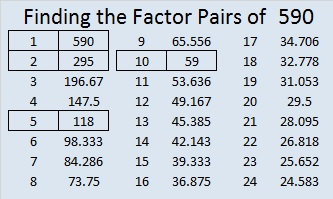# 590 and Level 5

3(20^2)/2 – 20/2 = 600 – 10 = 590. That means that 590 is the 20th pentagonal number.

590 is also the hypotenuse of the Pythagorean triple 354-472-590. Which factor of 590 is the greatest common factor of those three numbers? Hint: In this case the GCF is the difference between the middle number in the triple and either of the other two numbers.Print the puzzles or type the solution on this excel file: 10 Factors 2015-08-17

—————————————————————————————————

• 590 is a composite number.
• Prime factorization: 590 = 2 x 5 x 59
• The exponents in the prime factorization are 1, 1, and 1. Adding one to each and multiplying we get (1 + 1)(1 + 1)(1 + 1) = 2 x 2 x 2 = 8. Therefore 590 has exactly 8 factors.
• Factors of 590: 1, 2, 5, 10, 59, 118, 295, 590
• Factor pairs: 590 = 1 x 590, 2 x 295, 5 x 118, or 10 x 59
• 590 has no square factors that allow its square root to be simplified. √590 ≈ 24.2899—————————————————————————————————This site uses Akismet to reduce spam. Learn how your comment data is processed.